This node gives maximum freedom to create a linear composition between two terrains using a mask applied to the second terrain.

The principle is the same as the Composition with mask node, but the second terrain can be translated in the X and Y axes, scaled, and rotated before composing it with the first terrain.

To add a Full composition node, right click in the Graph Editor and select Create Node  > Terrain Composition  > Full composition using mask.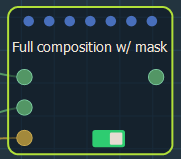Double click on the node to open its parameters: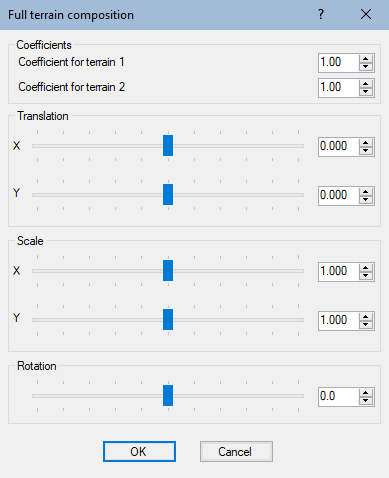The gizmo appears in the 3D View: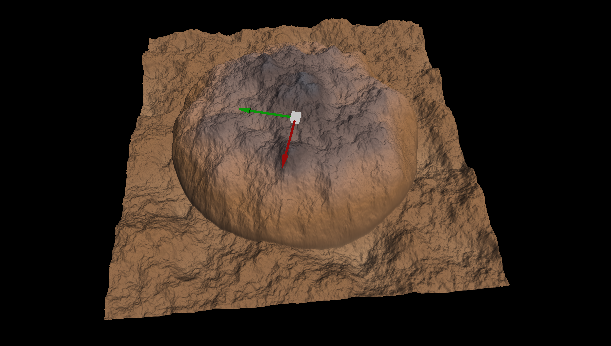If the size or resolution of the mask is not equal to that of the output terrain, the mask is automatically enlarged or reduced so that its size corresponds exactly to that of the output terrain.

Setting the coefficients

Edit the coefficients in the parameters dialog and see the terrain change in real time.

• Coefficient for the first terrain: Sets the coefficient by which the height of the first input terrain is multiplied.
• Coefficient for the second terrain: Sets the coefficient by which the height of the second input terrain is multiplied.

- When both coefficients are equal to 1, the result is identical to that produced by the Sum node.
- When the first coefficient is equal to 1 and the second is equal to -1, the result is identical to that produced by the Difference node.
- When both coefficients are equal to 0.5, the result is identical to that produced by the Average node.

Transforming the terrain

Use the sliders to set the translation, scale, and rotation values or click and drag either the center, the X axis, or the Y axis of the gizmo.

• Translation: Translates the second input terrain along the X or Y axis before composing it with the first terrain.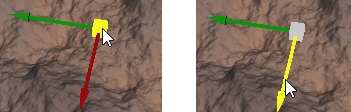The translation values are relative to the size of the output terrain:
- A value of 0 positions the center of the second input terrain at the center of the output terrain.
- An X value of -1 positions the center of the second input terrain on the left edge of the output terrain.
- A Y value of 1 positions the center of the second input terrain on the right edge of the output terrain.

• Scale: Sets the X axis or Y axis scale to be applied to the second input terrain before composing it with the first terrain.

Right-click on the terrain to change to the scale gizmo. Use the gizmo to set the scale values by clicking and dragging either the center, the X axis, and the Y axis of the gizmo: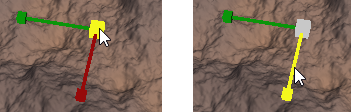The default scale value is 1, which means that the second input terrain retains its default size. This is not the case for the Terrain composition node where the second input terrain is automatically scaled so that its size corresponds to that of the output terrain.

• Rotation: Sets the angle in degrees to rotate the second input terrain around its center before composing it with the first terrain.

Right-click on the terrain to change to the rotate gizmo. Drag the mouse when the gizmo is highlighted in yellow to change the angle of rotation.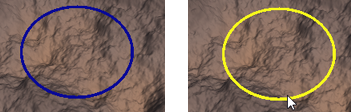Video tutorial

Watch our Composition using mask nodes video tutorial, which explains how the Full composition using mask node and the Composition using mask node work.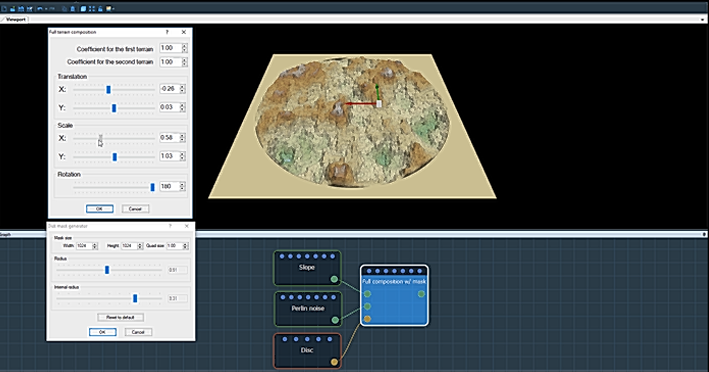Parameters

Parameter Use
Coefficient for the first terrain The coefficient by which the height of the first input terrain is multiplied
Coefficient for the second terrain The coefficient by which the height of the second input terrain is multiplied
Translation - X Translation along the X axis to be applied to the second input terrain before composing it with the first terrain
Translation - Y Translation along the Y axis to be applied to the second input terrain before composing it with the first terrain
Scale - X The X scale to be applied to the second input terrain before composing it with the first terrain
Scale - Y The Y scale to be applied to the second input terrain before composing it with the first terrain
Rotation The angle in degrees to rotate the second input terrain around its center before composing it with the first terrain